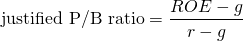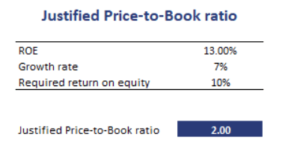# Justified Price-to-book multiple

The justified price-to-book multiple or justified P/B multiple is a P/B ratio based on the company’s fundamentals. The justified P/B ratio is based on the Gordon Growth Model. It uses the sustainable growth relation and the observation that expected earnings per share equal book value times the return on equity.

On this page, we provide the justified price-to-book formula, interpret the ratio, and implement a justified P/B multiple example in Excel. The spreadsheet is available at the bottom of the page.

## Justified P/B multiple formula

Let’s start by discussing the ratio. The formula for the justified P/B equalswhere ROE is the return on equity, g is the sustainable growth rate (= ROE*b, where b is the retention rate), and r is the required rate of return on equity. This formula is considerably easier than the related justified P/E multiple.

## Justified P/B multiple interpretation

We can draw several conclusions from the formula on the relationship between a company’s fundamentals and the price-to-book of the company. In particular, we can see that the P/B increases as ROE increases. Also, the higher the spread between ROE and r, all else equal, the higher the P/B ratio.

This makes sense. The ROE is the return on the firm’s investments while r is the required return. Thus, the more projects a company can realize that generate more than the required return on equity, the more valuable the company is. This increases the market value of the company.

## Justified price-to-book example

Using forecasts on g, ROE, and r, we can calculate the justified ratio. We can then compare this value to the actual P/B ratio of the company. If the justified P/B is higher, then the company is undervalued. Similarly, if the justified P/B is lower than the actual P/B multiple, then the company is overvalued based on this approach.

The following table illustrates the calculation of the ratio using the inputs discussed above. the spreadsheet used to create the table can be downloaded at the bottom of the page.## Summary

We discussed the justified P/B ratio, a ratio based on the Gordon Growth model that provides a P/B ratio that is justified based on the company’s fundamentals.